# Python Program to Count Number of Character in String

In this article, I've created some programs in Python, that counts number of characters available in a string entered by user. Here are the list of programs:

• Count Particular Character in a String. Both character and string must be entered by user
• Count lowercase characters in a string
• Count uppercase characters in a string
• Count all characters in a string

## Count Occurrence of a Character in a String

To count the occurrence of, or to find frequency of, a particular character in a string in Python, you have to ask from user to enter a string and a character both, then find and print the frequency of given character in given string as shown in the program given below:

The question is, write a Python program to count occurrence of a character in a string. Here is its answer:

```print("Enter the String:")
text = input()
print("Enter the Character:")
char = input()
textLen = len(text)
sum = 0
for i in range(textLen):
if char==text[i]:
sum = sum+1
print("\nOccurrence of Given Character is:")
print(sum)```

Here is its sample run: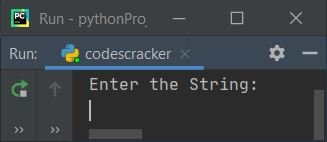Now supply the input say codescracker as string and then e as character to count. Press `ENTER` key to count and print the occurrence of e in given string as shown in the snapshot given below: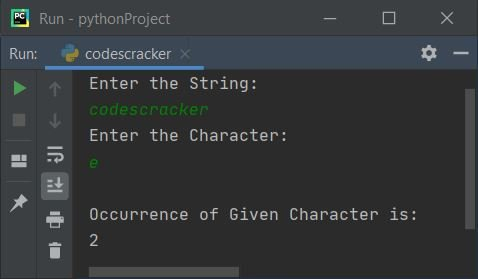The dry run of above program with same user input as provided in above sample run, goes like:

• Initial values, text = "codescracker" (entered by user), char = "e" (entered by user)
• Then len(text) or len("codescracker") or 12 gets initialized to textLen, and sum=0
• The range() method returns a sequence of values. By default, starts with 0, increments by 1, and continues before the value given as its argument (if only one argument is given)
• That is, i's initial value is 0 at first evaluation of for loop, since 0 is less than textLen's value (that is 12), therefore condition evaluates to be true, and program flow goes inside the loop
• Inside the loop, we've compared the value of char to the character at ith index of text every time. When a match found, then the value of sum gets incremented.
• That is, the condition (of if) char == text[i] or "e" == text or "e" == "c" ("c" is very first character of given string) evaluates to be false, therefore program flow does not goes inside this if's body. And the value of sum does not gets incremented
• Now the value of i gets incremented by 1. So i=1. Since 1 is less than 12 (textLen's value), therefore condition again evaluates to be true, and program flow again goes inside the loop
• Again the condition char == text[i] or "e" == text or "e" == "o" evaluates to be false
• Now i's value again gets incremented. So i=2 now. This process continues before i's value becomes equal to 12
• When the value at char gets matched with value at ith index of text, then we've incremented the value of sum
• In this way, after exiting from the loop, the variable sum holds the value that shows the frequency of character "e" in "codescracker"
• So just print the value of sum as frequency of character in string

## Find Frequency of Particular Character in String using count()

This program find and prints frequency of a particular character (entered by user) in a string (entered by user) using count() method.

```print("Enter the String:")
text = input()
print("Enter the Character to Count:")
char = input()
val = text.count(char)
print("Occurrence of Given Character is: ")
print(val)```

Here is its sample run with user input this is codescracker as string and then c as character to count: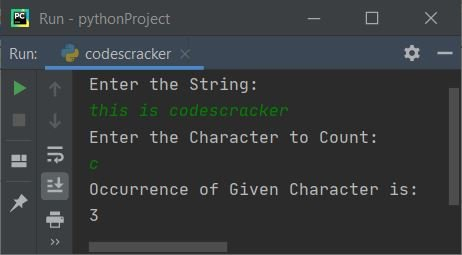#### Modified Version of Previous Program

This is the modified version of previous program. The end is used to skip inserting an automatic newline using print(). The \" is used to print ". And the str() method converts any type of value to a string type.

```print(end="Enter the String: ")
text = input()
print(end="Enter the Character: ")
char = input()
val = text.count(char)
print("\n\"" + char + "\" occurs " + str(val) + " time(s)")```

Here is its sample run with user input codescracker as string and e as character:## Count Lowercase Characters in String

This program counts only lowercase characters (a-z) in a given string by user, using for loop and indexing:

```print(end="Enter the String: ")
text = input()
textLen = len(text)
sum = 0
for i in range(textLen):
if text[i]>='a' and text[i]<='z':
sum = sum+1

print("\nLowercase Characters = " + str(sum))```

Here is its sample run with user input, CodesCracker: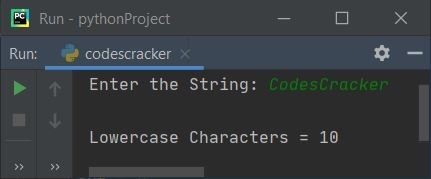## Count Uppercase Characters in String

This program count and prints only uppercase characters (A-Z) in a string entered by user at run-time:

```print("Enter the String: ", end="")
text = input()
textLen = len(text)
sum = 0
for i in range(textLen):
if text[i]>='A' and text[i]<='Z':
sum = sum+1

print("\nUppercase Characters = " + str(sum))```

Here is its sample run with same user input as of previous program's sample run: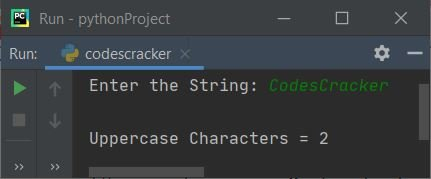## Count Total Number of Characters in String

Now this program counts all characters in a string. It doesn't cares about what the character is like lowercase, uppercase, space, digit, etc., everything gets counted:

```print("Enter the String: ", end="")
text = input()
textLen = len(text)
sum = 0
for i in range(textLen):
sum = sum+1

print("\nTotal Characters = " + str(sum))```

Here is its sample run with user input, Welcome to CodesCracker:#### Same Program in Other Languages

Python Online Test

« Previous Program Next Program »